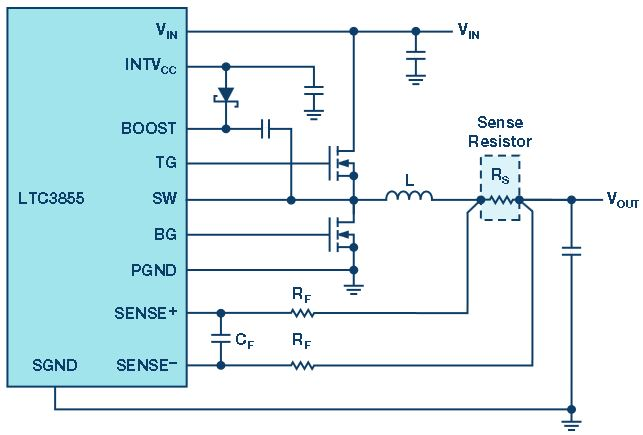# Switch Mode Power Supply Current Sensing—Part 1: The Basics

Current-mode control is widely used for switching mode power supplies due to its high reliability, simple loop compensation design, and simple and reliable load sharing capability. The current sense signal is an essential part of a current-mode switch mode power supply design; it is used to regulate the output and also provides overcurrent protection. Figure 1 shows the current sensing circuit for an LTC3855 synchronous switching mode step-down power supply. The LTC3855 is a current-mode control device with cycle-by-cycle current limiting. The sense resistor RS monitors the current.Figure 1. The switch mode power supply current sense resistor (RS).

Figure 2 shows a scope image of the inductor current for two cases: in one case, with a load that the inductor current is capable of driving (red line), and in the second case, where the output short circuited (purple line).Figure 2. LTC3855 current limit with foldback example, as seen on a 1.5 V/15 A rail.

Initially, the peak inductor current is set by the inductor value selected, the power switch ON time, the input and output voltages of the circuit, and the load current (signified by “1” on the plot). When the short circuit is applied, the inductor current quickly ramps upward until it hits the current limit at the point where RS × IINDUCTOR (IL) equals the maximum current sense voltage—protecting both the device and downstream circuitry (signified by “2” on the plot). After that, the built-in current foldback limit (number “3” on the plot) further reduces the inductor current to minimize thermal stress.

Current sensing also serves other purposes. It allows accurate current sharing in a multiphase power supply design. With lightly loaded power designs, it can be used to increase efficiency by preventing reverse current flow (reverse currents are currents that flow the opposite way through the inductor, from output to input, which may be undesirable or even destructive in some applications). In addition, when a multiphase application is lightly loaded, current sensing can be used to reduce the number of phases needed, which increases circuit efficiency. For loads that require a current source, current sensing can turn the power supply into a constant current source for applications such as LED driving, battery charging, and driving lasers.

In Part 2 of this series, “Where to Place the Current Sense Resistor,” we cover in which leg of the circuit to place the current sense resistor and how it affects operation.

### Software

#### LTspice

LTspice® software is a powerful, fast, and free simulation tool, schematic capture, and waveform viewer with enhancements and models for improving the simulation of switching regulators.